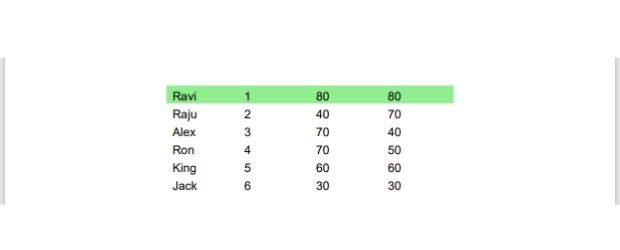# PDF file Table from Pandas DataFrame

PDF PandasAdding pandas dataframe created from SLQite or MySQL database table to PDF file by Python ReportLab

Our main file table_pandas.py ( Copy source code at the end of this page ) only reads the data as a list ( my_data ) from the data file.

Inside the table_pandas.py we import the my_data variable containing data as a list.
``````from reportlab.pdfgen import canvas
my_path='G:\\My drive\\testing\\pypdf2\\my_pdf.pdf'
from reportlab.lib.units import inch
from reportlab.lib.pagesizes import letter
from reportlab.platypus import SimpleDocTemplate
from reportlab.platypus.tables import Table,TableStyle,colors
from table_pandas_data import my_data # import the data
my_doc = SimpleDocTemplate(my_path,pagesize=letter)

c_width=[1*inch] # width of the columns
t=Table(my_data,colWidths=c_width,repeatRows=1)
t.setStyle(TableStyle([('FONTSIZE',(0,0),(-1,-1),12),
('BACKGROUND',(0,0),(-1,0),colors.lightgreen),('VALIGN',(0,0),(-1,0),'TOP')]))
elements=[]
elements.append(t)
my_doc.build(elements)``````

## Creating DataFrame

Inside our table_pandas_data.py we will create the dataframe by using one dictionary as source of data.
``````import pandas as pd
my_dict={'NAME':['Ravi','Raju','Alex','Ron','King','Jack'],
'ID':[1,2,3,4,5,6],
'MATH':[80,40,70,70,60,30],
'ENGLISH':[80,70,40,50,60,30]}
df = pd.DataFrame(data=my_dict) # dataframe
my_data=df.values.tolist() # create a list using Dataframe``````
Here the last line creates our required data source my_data as a list.

## Using Database table as datasource

In above code the variable my_data can be created by using data ( value ) from different data sources. Let us try SQLite database student as source for creating this variable. As SQLite is a file based database, the sample file with student table can be downloaded.

We used try except error handling to show error message.

``````import pandas as pd
from sqlalchemy import create_engine
from sqlalchemy.exc import SQLAlchemyError
db_file='G:\\My drive\\testing\\my_db.db'
file1='sqlite:///'+ db_file
my_conn = create_engine(file1)
#my_conn = create_engine("mysql+mysqldb://id:pw@localhost/my_tutorial")
try:
df = pd.read_sql("SELECT * FROM student",my_conn)
my_data=df.values.tolist()   # add rows as list.
my_data.insert(0,df.columns) # add the columns at first row
except SQLAlchemyError as e:
error = str(e.__dict__['orig'])
print(error)``````

## Using MySQL database

In above code we can create the connection object my_conn by using this connection string for MySQL.
``````from sqlalchemy import create_engine

table_pandas.py

table_pandas_data.py

Subscribe to our YouTube Channel here

## Subscribe

* indicates required
Subscribe to plus2netplus2net.com

Post your comments , suggestion , error , requirements etc here